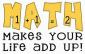# find the particular solution?

1 post / 0 new
Juny Geraldizo ...find the particular solution?

[(1-xy)^-2]dx + [y^2-x^2(1-xy)^-2]dy=0 ;when x=1, y=1

• Mathematics inside the configured delimiters is rendered by MathJax. The default math delimiters are $$...$$ and $...$ for displayed mathematics, and $...$ and $...$ for in-line mathematics.